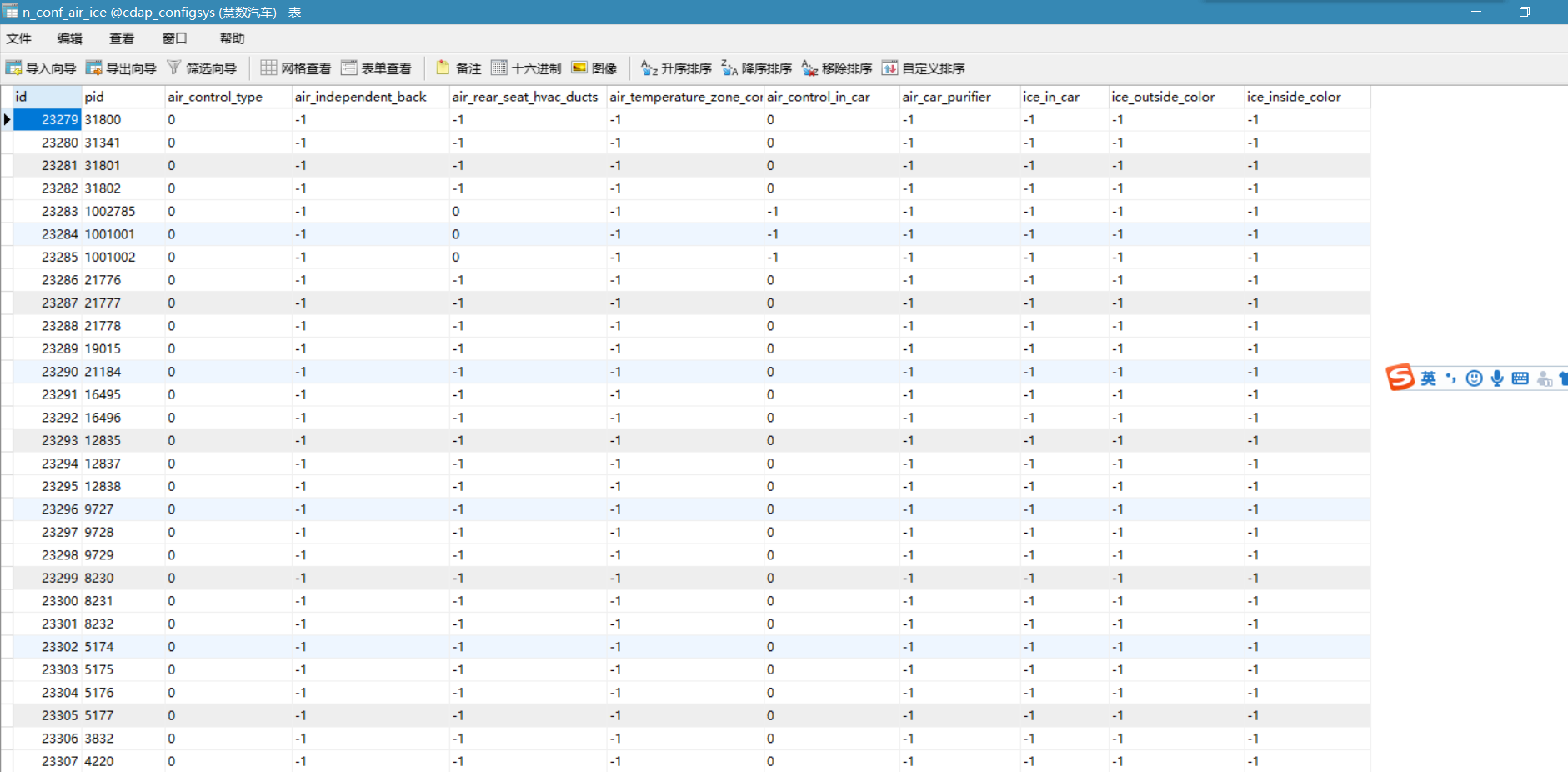mysql 统计每列即每个字段下的0,1，-1的个数6个回答

SELECT COUNT(CASE WHEN (A.air-control-type=0) THEN 0 END),
COUNT(CASE WHEN (A.air-control-type=1) THEN 0 END),
COUNT(CASE WHEN (A.air-control-type=-1) THEN 0 END),
COUNT(CASE WHEN (A.air-independent-back=0) THEN 0 END),
COUNT(CASE WHEN (A.air-independent-back=1) THEN 0 END),
COUNT(CASE WHEN (A.air-independent-back=-1) THEN 0 END)
FROM T_TABLE AS Sazuoyayun select air-control-type,count(air-control-type) num from count1 group by air-control-type; select air-independent-back,count(air-independent-back) num from count1 group by air-independent-back;select
field_name,
field_value,
field_value_count
from
(
select 'c1' as field_name , c1 as field_value, count(1) field_value_count as cnt from my_table group by c1
union all
select 'c2' as field_name , c2 as field_value, count(1) field_value_count as cnt from my_table group by c2
union all
select 'c3' as field_name , c3 as field_value, count(1) field_value_count as cnt from my_table group by c3
) tmp

SELECT SUM( DECODE(air-control-type,0,1,0)) air-control-type0,
SUM( DECODE(air-control-type,1,1,0)) air-control-type1,
SUM( DECODE(air-control-type,-1,1,0)) air-control-type-1 ,.....FROM TABLEqq_29127157 回复姜某人: SELECT SUM(case when air-control-type = 1 then 1 else 0 end) air-control-type1, SUM(case when air-control-type = 0 then 1 else 0 end) air-control-type0, SUM(case when air-control-type = 0 then 1 else 0 end) air-control-type-1,... FROM TABLENAMESELECT air-control-type,COUNT(air-control-type),air-independent-back,COUNT(air-independent-back) FROM tablename GROUP BY air-control-type,air-independent-backazuoyayun 这个是不行的，多个分组，获取到的数据不对select sum(case when air-control-type = 0 then 1 else 0 end) zero_count,
sum(case when air-control-type= 1 then 1 else 0 end) one_count from table;

select count(air-control-type = 0 or null) typeZeroCount, count(air-control-type = 1 or null) typeOneCount, count(air-control-type = -1 or null) typeNegativeCount from table;